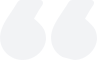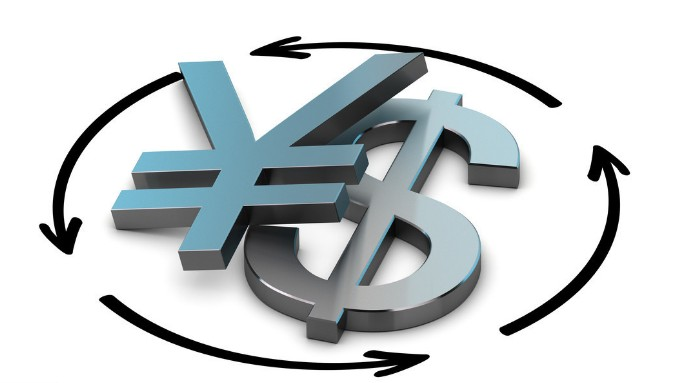# Is foreign exchange rate volatility a foreign exchange rate? What is the difference between the two?

2023-06-05Summary:

Foreign exchange rate refers to the exchange rate between two currencies, while foreign exchange rate volatility refers to the fluctuation of this exchange rate over a period of time. The two sound similar, although both are related to exchange rates, they are different in many places.

Is foreign exchange rate volatility a foreign exchange rate?

Foreign exchange rate volatility is not a foreign exchange rate, but an indicator used to measure the magnitude of changes in the foreign exchange rate. The foreign exchange rate is the exchange ratio between two currencies, while the foreign exchange rate volatility refers to the fluctuation of this rate over a period .

The volatility of foreign exchange rates is usually expressed using statistical indicators such as standard deviation or variance. It can reflect the degree of uncertainty in the market regarding future exchange rate trends, and higher volatility means that the market expects a wider range of exchange rate fluctuations. Therefore, the volatility of foreign exchange rates is very important for foreign exchange market participants, which can help them formulate risk management strategies and investment decisions.What is the difference between the two?

The difference between foreign exchange rate volatility and foreign exchange rate is that they measure different objects.

Foreign exchange rate refers to the exchange rate between two currencies, which is how much one currency can be exchanged for another currency. It is usually represented by a fixed number, such as 6.5 RMB for 1 US dollar.

The volatility of foreign exchange rates is an indicator used to measure the magnitude of changes in foreign exchange rates, which can reflect the degree of uncertainty in the market regarding future exchange rate trends. It is usually calculated using statistical methods and expressed as a percentage or numerical value. For example, if the volatility of a currency pair is 10%, it means that the exchange rate of the currency pair may fluctuate by 10% over a period of time.

Therefore, foreign exchange rate is a specific currency exchange rate, while foreign exchange rate volatility is an indicator of the fluctuation of this exchange rate over a period of time.

【 EBC Platform Risk Reminder and Disclaimer 】: There are risks in the market, and investment needs to be cautious. This article does not constitute investment advice.### What is the status of the volume-price relationship?

The volume-price relationship is a key stock market indicator, revealing the correlation between trading volume and stock prices. Analyzing these changes helps investors understand market activity and potential trend reversals.

2023-12-01### What is delisting?

Delisting removes a stock from public trading. It's either voluntary or mandatory, due to violations, financial issues, mergers, etc.

2023-12-01### What does the exchange rate mean?

The exchange rate, reflecting the relative values of two currencies, is influenced by factors like currency supply and demand, balance of payments, economic growth rate, interest rates, monetary policy, and inflation.

2023-11-29